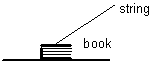Teaching Physics with the Physics Suite Edward F. Redish

Pulling a book

 A string is tied to a book and pulled at an angle as shown at the right. The book remains in contact with the table and does not move.1. How do the forces exerted on the book in this case compare to the forces exerted on the book when the string is not present? Describe the force(s) that are the same (meaning same type of force and same magnitude) in both cases. Describe the force(s) that change when the string is pulled.

2. The string is pulled hard enough that the book begins to slide. After a short start up period, the book is moving at a constant velocity. At this instant of time, the tension in the string is 5 N and the angle the string makes with the horizontal is 30†. If the book has 1200 pages and a mass of 1.5 kg, find the coefficient of friction between the book and the table. (If needed, you may approximate the density of water by 1 gm/cm3 and the gravitational field strength by 10 N/kg.)

3. Draw a free-body diagram for the book. Label each of the forces exerted on the book being sure to specify the type of force and who is causing the force as well as who is feeling the force.

4. In part (b) [the question about the coefficient of friction], was a free-body diagram useful (or would it have been useful) in helping you think through the problem? Or is this one of those cases in which drawing the free-body diagram was a separate problem designed to help the grader see if you understand what forces are present?

Not finding what you wanted? Check the Site Map for more information.